# Coin Sorter

Image Processing Based Coin Sorter
by Yidan Wang (yw482) and Zhihao Liu (zl385)

## Introduction

A coin sorting machine is a machine that can sort a random collection of coins into seperate bins. We intended to design a coin sorter that could sort the four most common coins in the U.S.: Quarter(¢25), Dime(¢10), Nickel(¢5) and Penny(¢1). We were interested in using image processing technology to recognize and sort these four common U.S. coins. Our system was built with Raspberry Pi, OpenCV and some machine learning algorithm. Raspberry Pi was used to run our program and control all mechanical modules. OpenCV was used to find the coin within a picture taken by Pi Camera. Machine learning was used to identify the color of coins to better distinguish the coins that are similar sizes but different colors. We also provided an user interface on PiTFT to let user interact with the program, display the quantity of each collected coin and total amount of collected money value.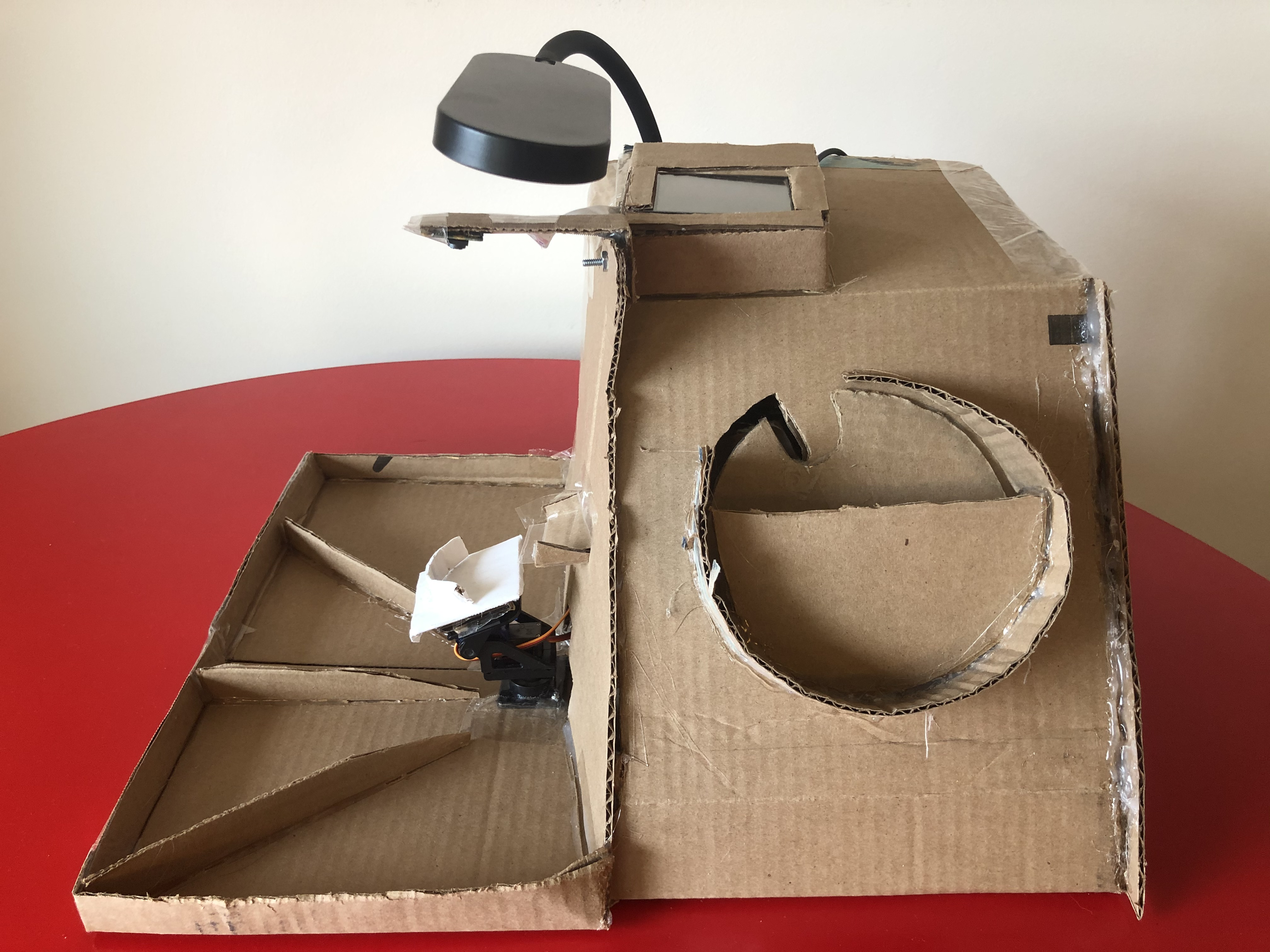## Project Objective:

• The machine can distinguish Quarter(¢25), Dime(¢10), Nickel(¢5) and Penny(¢1) with high accuracy.
• The machine can display current total value and the quantity of each type of coin.
• User is able to start, reset and quit the program.

## Design and Testing

### Process Flow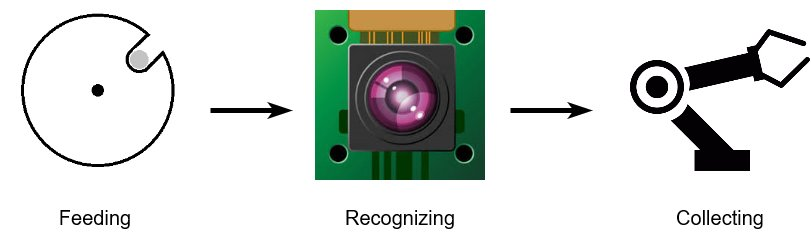#### Feeding

We designed a spinning plate that has a coin slot. The spinning plate with a coin slot is only able to hold one coin a time from a random collection of coins. The spinner will rotate and the coin in the slot will be dropped onto a slope surface. The coin will slide down the slope surface onto a platform. The platform is used to hold the coin for taking pictures with Pi Camera.

#### Recognizing

Once a coin is held on the platform. The program will stop the spinning plate and take several pictures of the coin with Pi Camera. After the picture is taken, the program will run our image processing and machine learning algorithm to recognize if the coin is Quarter(¢25), Dime(¢10), Nickel(¢5) or Penny(¢1). The program will also update the display on PiTFT to show total value and the quantity of each coin.

#### Collecting

The collecting module was built with a two degrees of freedom robot arm. Once a coin is recognized by our algorithm, the program will run a robot arm control program to drop the coin into corresponding bins. We have four bins for the four types of coins.

### Hardware Design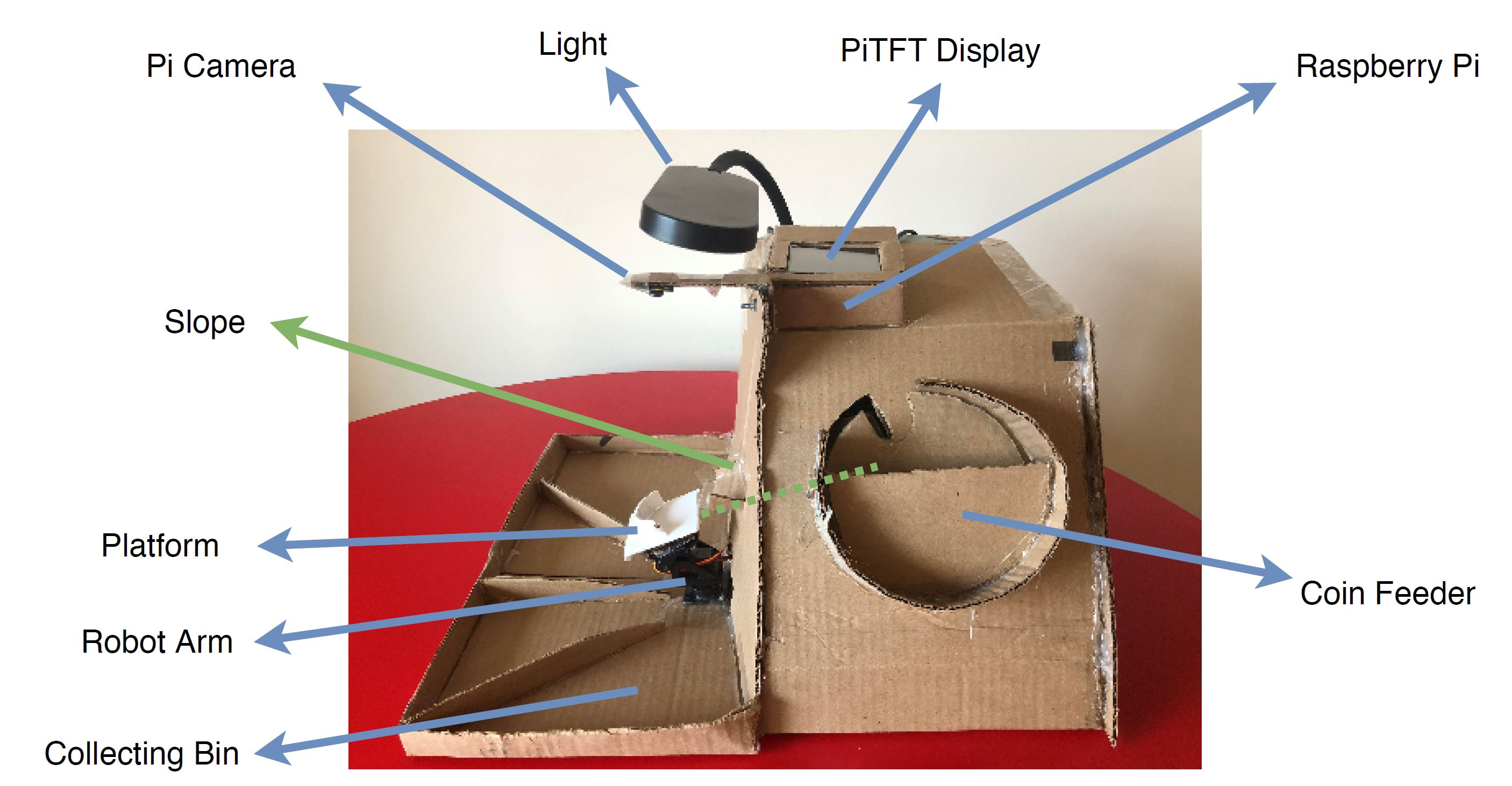We made the hardware structure mainly with cardboard. We started our project by designing the Coin Feeder. The Coin Feeder is made of cardboard. We cut a piece of cardboard into a circular plate and cut a coin slot on the plate. The center of plate is adhering to a continuous servo. When the plate is rotated by the servo, it is able to pick only one coin in the slot. Once the spinning plate rotates to a certain position, the coin will fall onto a slope. The slope is made of cardboard and installed with an angle. The surface of the slope is covered with clear tape to decrease friction so that a coin can easily slide down the slope. Once the coin slides onto the platform, which is designed to hold the coin in an appropriate position, the Pi Camera will take several pictures to do coin recognition. We installed an extra light above the Pi Camera to increase the picture quality which dramatically increases the accuracy of our coin recognizing algorithm. Since the platform is attached to the robot arm, our program will control the robot arm to drop the coin into corresponding collecting bins.

#### Coin Feeder

The Coin Feeder is driven by a Parallax Continuous Rotation Servo (#900-00008). We used a GPIO pin to generate PWM signal to rotate the servo. The servo is powered 4 AA batteries. We set the pulse of the PWM signal to 1.54ms to let the servo rotate counter-clockwise. The value of 1.54ms is determined after we had done several rotation speed tests to achieve the best feeding rate.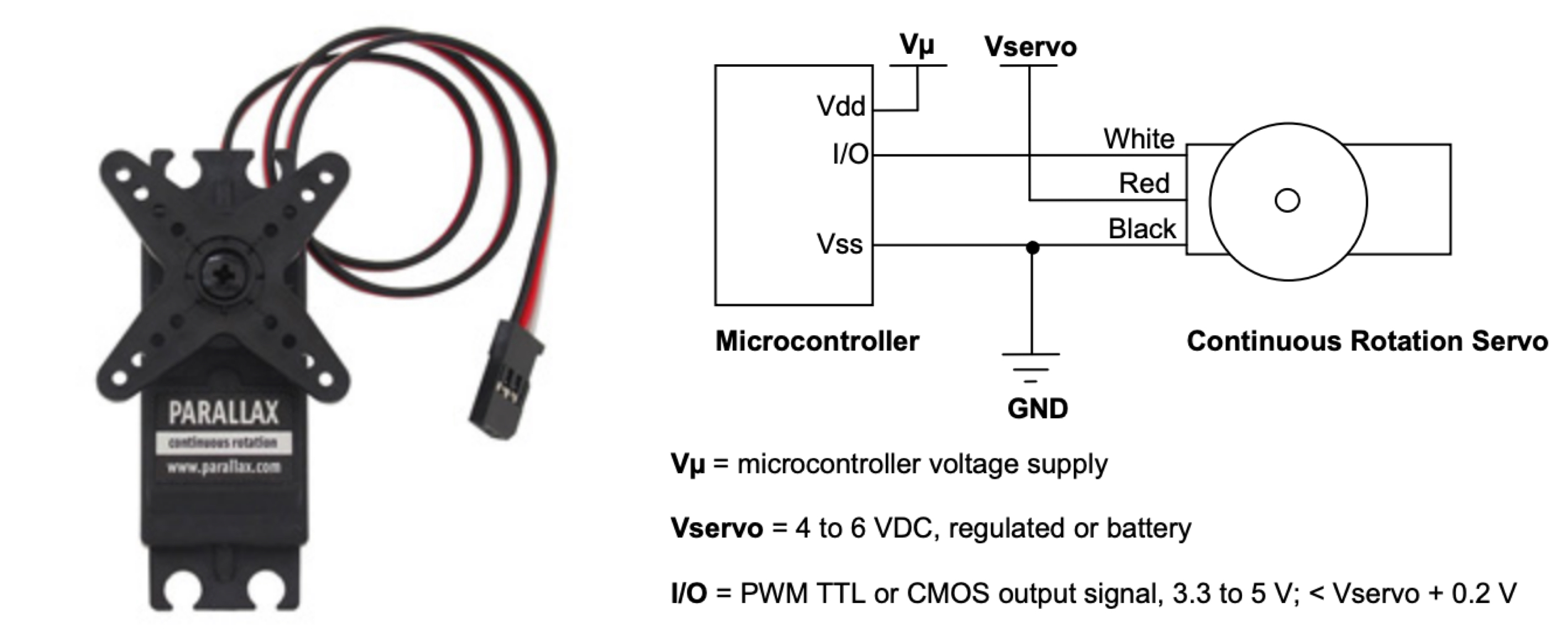#### Robot Arm

The Robot Arm is driven by a Pan-Tilt HAT made by Waveshare. This device is driven by I2C interface. In order to use I2C interface, we enabled the I2C kernel module in Raspberry Pi Software Configuration Tool (raspi-config). Then, we found the driver for this device on the manufacturer's website. The driver is PCA9685, an I2C-bus controlled 26-channel LED controller, 12-bit resolution PWM output. We used the driver to design our control program for the robot arm. We designed the control program to be able to drop coins to four directions, which is facing four collecting bins. The program can be easily run by giving it the direction.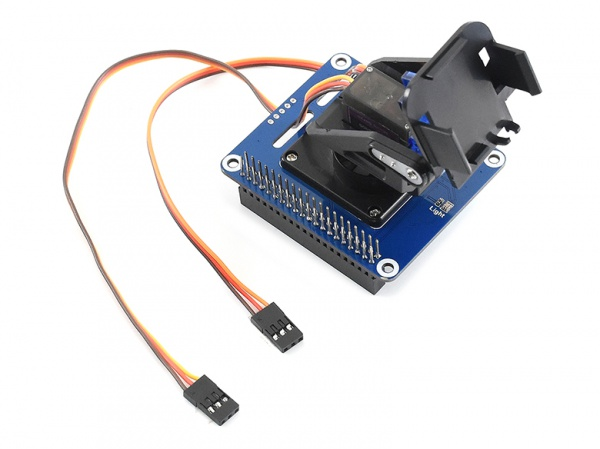### Software Design

#### Overall design

We created a single Python class called CoinSorter for running our coin sorter machine. Here we will explain all the key attributes and member methods of this class.

Key attributes
• self.penny, self.nickel, self.dime, self.quarter: integer attributes to record the number of each type of coins we have so far.
• self.robot: a wrapper class to control the robot arm (a Pan-Tilt HAT) that moves coins to different bins
• self.spinner: a GPIO.PWM object to control the movement of the servo of the coin spinner
• self.camera: a PiCamera Python object to control the Raspberry Pi camera
• self.model_penny_or_dime: a pre-trained machine learning model loaded from hard disk to distinguish penny and dime. It turns out this model also performs well in distinguishing penny and non-penny (although we only use penny and dime sample pictures to train this model)
• self.die: indicate whether we will stop sorting and quit the program
Key member functions (the self argument is neglected below):
• start_spinner(), stop_spinner(): start/stop the coin spinner
• take_snapshot(): take a single picture of the platform using PiCamera and return it
• take_continuous_snapshots(): a generator which produces a stream of pictures of the platform using PiCamera
• reset(): reset the coin count for each coin type
• start_job(): core function to run the coin sorter machine. It’s basically running a persistent loop to detect coin types (if there is one) and put them into the right bin.
• increment_type_count(coin_type): increment the count of the corresponding coin type
• get_coin_type(img): core function to predict a coin type (if there is one) given a picture taken from the PiCamera
• put_coin(coin_type): method to instruct the robot arm to put the coin into the right bin
• shutdown(): cleanup method to reset robot arm position and shut it down, stop the spinner and close the camera

#### Workflow diagram for the CoinSorter class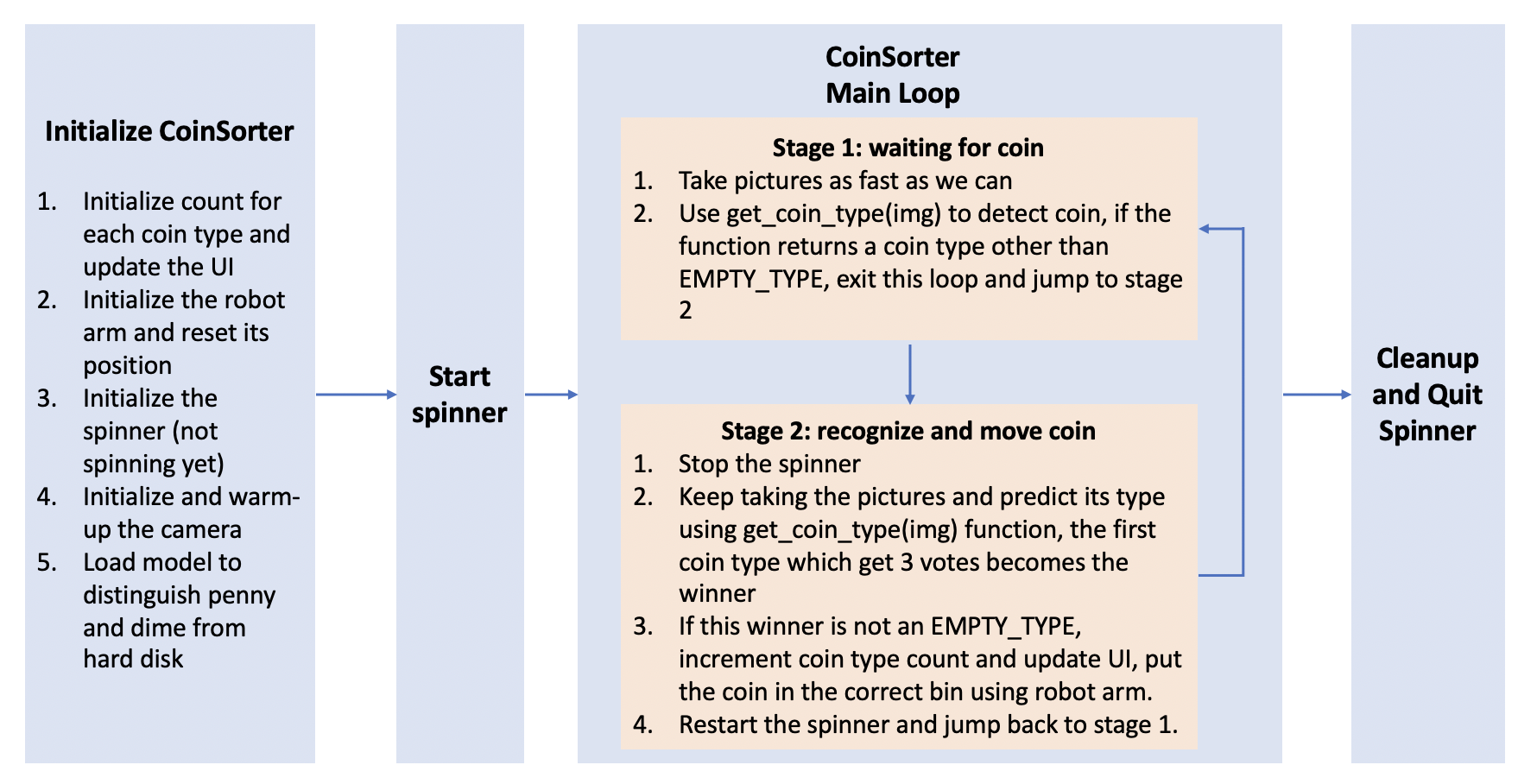Above is the main workflow for the coin sorting machine. Several points worth mentioning here:

• We have two threads for running the coin sorting machine. The main thread will constantly check user input on the touchscreen of the PiTFT and make responses accordingly (update UI, send signals to the CoinSorter object). The other thread will be used to run the start_job() method of the CoinSorter Object.
• We introduce voting for detecting types of coins because our algorithm cannot guarantee 100% accuracy. As a result, in order to increase the confidence of our judgement, we will take a stream of pictures (possibly with differences in lighting conditions and small jitters in other parameters) and make a prediction and vote for the final winner.

#### Method to detect coin type

To detect coin type, we need to take a look at both size and color. Among four types of US coins, penny is brass while others are silver. In terms of size, quarter is the biggest, nickel comes the second, and follows dime and penny, which are pretty similar in size. We have separate models to detect size and color, which are explained in detail below.

##### Models to detect coin size
###### 1) Model 1: Take image difference

Everytime we take a new snapshot, we first crop both images so that only the area of the platform is kept. We then convert both images to grayscale and use structural_similarity() function from skimage.metrics to compare them. Using this method, we are able to produce an image with the same dimension that contains structural similarity information between the two images. Specifically, if the new snapshot taken contains a coin, all pixels within the coin area will be emphasized in the output image because those are considered significantly different than their corresponding pixels in the empty image. The next step is to find parameters of all the circles (if there is any) in this output image using the Hough transform. We have a handy function called HoughCircles() in cv2 to help us achieve this goal. After we get the radius of the circle, we could use some predefined threshold values to help us predict coin types.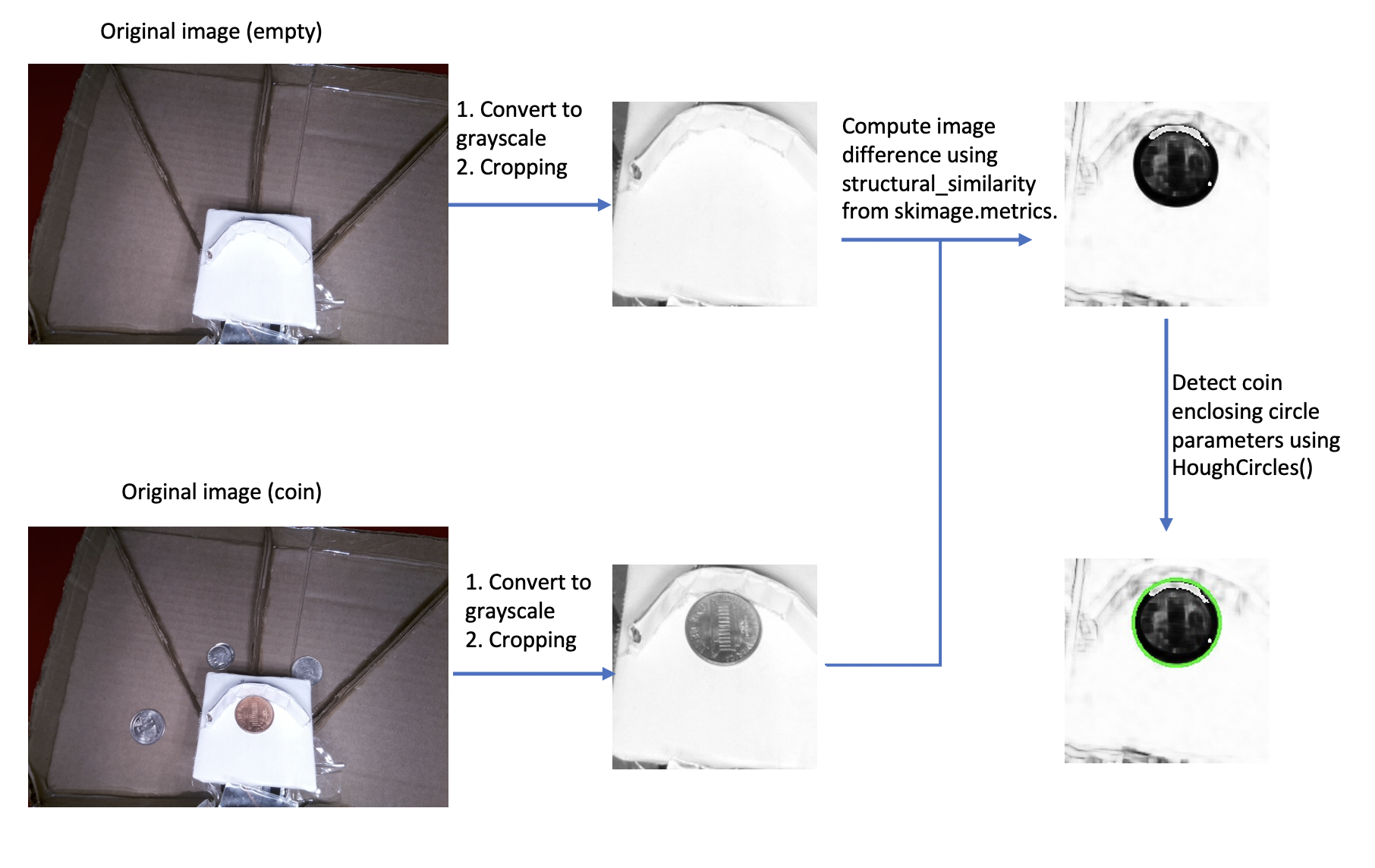###### 2) Model 2: Run HoughCircles() directly on cropped image

This model is more straightforward than the first model mentioned above. It turns out the prediction accuracy of it is also better so we adopt this model in the final version. The model does not have any overhead of caching images. Everytime we take a new snapshot, we just crop it to get the platform area and run HoughCircles() method directly on the grayscale version of the platform image. After we get the radius of the circle, we use the same predefined threshold values to help us predict coin types.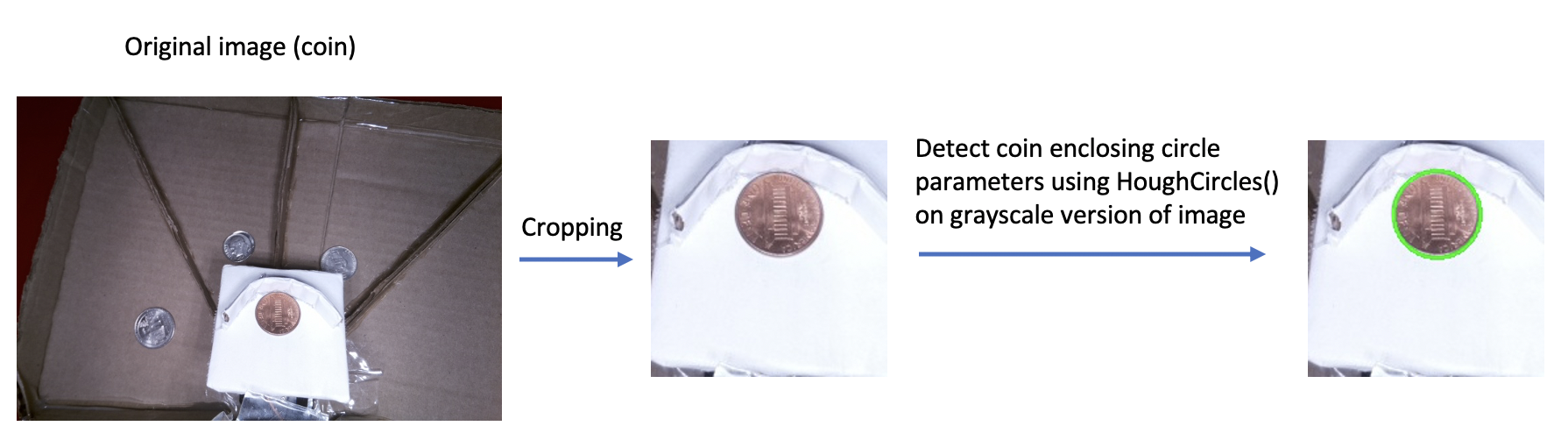##### Model to detect coin color

Here we present a model to help us distinguish penny (brass) and non-penny coins (silver). The idea here is very simple. Everytime we find a coin in the platform, we will find the square area which perfectly covers the coin, then we will use the calcHist() method from cv2 to calculate histogram information for pixels within the coin (we will create a cv2.circle() object to serve as the mask). The histogram has 8 bins on all 3 channels, as a result, we will get a 8*8*8 3-dimensional histogram. We normalize this histogram and flatten it to get a 1-d vector of 512 length. We then use a pre-trained model to predict the coin type taking this vector as input. The pre-trained model is a MLPClassifier but we can also pick any traditional machine learning classifier we want (logistic regression, svm, etc. It turns out they have similar performance on this task). We have made several hundred penny and non-penny pictures and train this model using the same process mentioned above to generate feature vectors. The model yielded a surprising prediction accuracy of 100% (It makes sense because the brass color and silver color should have significant difference in their color histograms)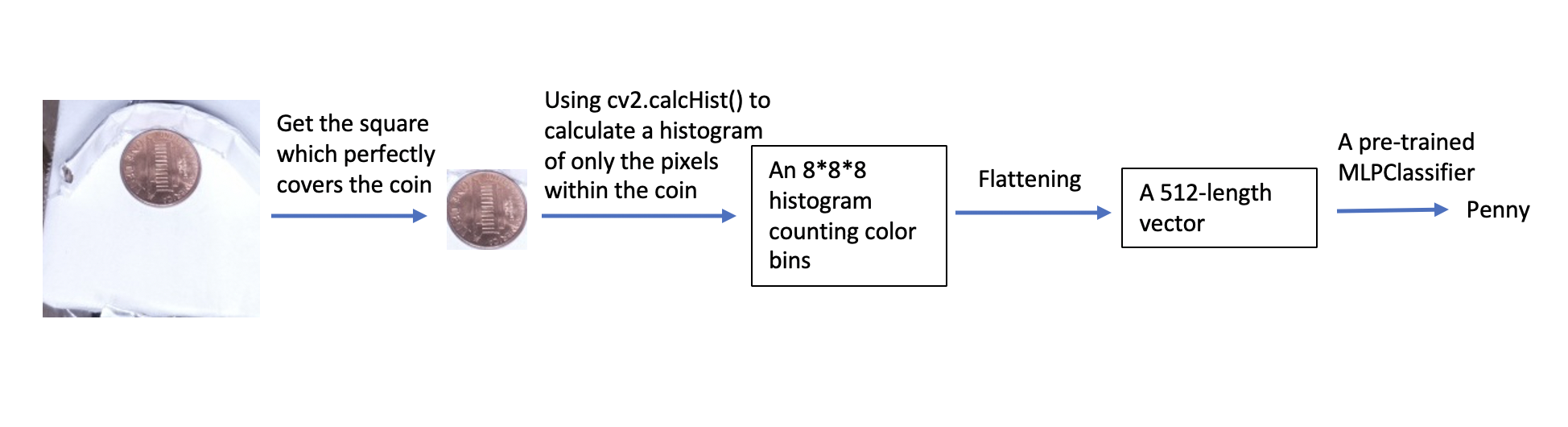##### Coin type prediction steps

Combining the size model (the second model) and color model above, we designed a pipeline to help us predict coin type as shown in the graph below. Notice that this is a single round of prediction, in order to ensure higher confidence, we will take multiple pictures and predict multiple times until we get 3 votes for any coin types. The final prediction model works really well with a 95% prediction accuracy approximately.#### UI Interface design

We used Python’s pygame library to help us design the user interface. This will be running as the main thread to detect any user input to the PiTFT touchscreen. The UI interface will keep counts of 4 different types of coins and the total amount in dollars. Also, it will have 3 buttons: a start button for the user to start the coin sorter, a reset button to reset the amount to 0.0 and a quit button to stop the coin sorter’s mechanical parts and exit the program.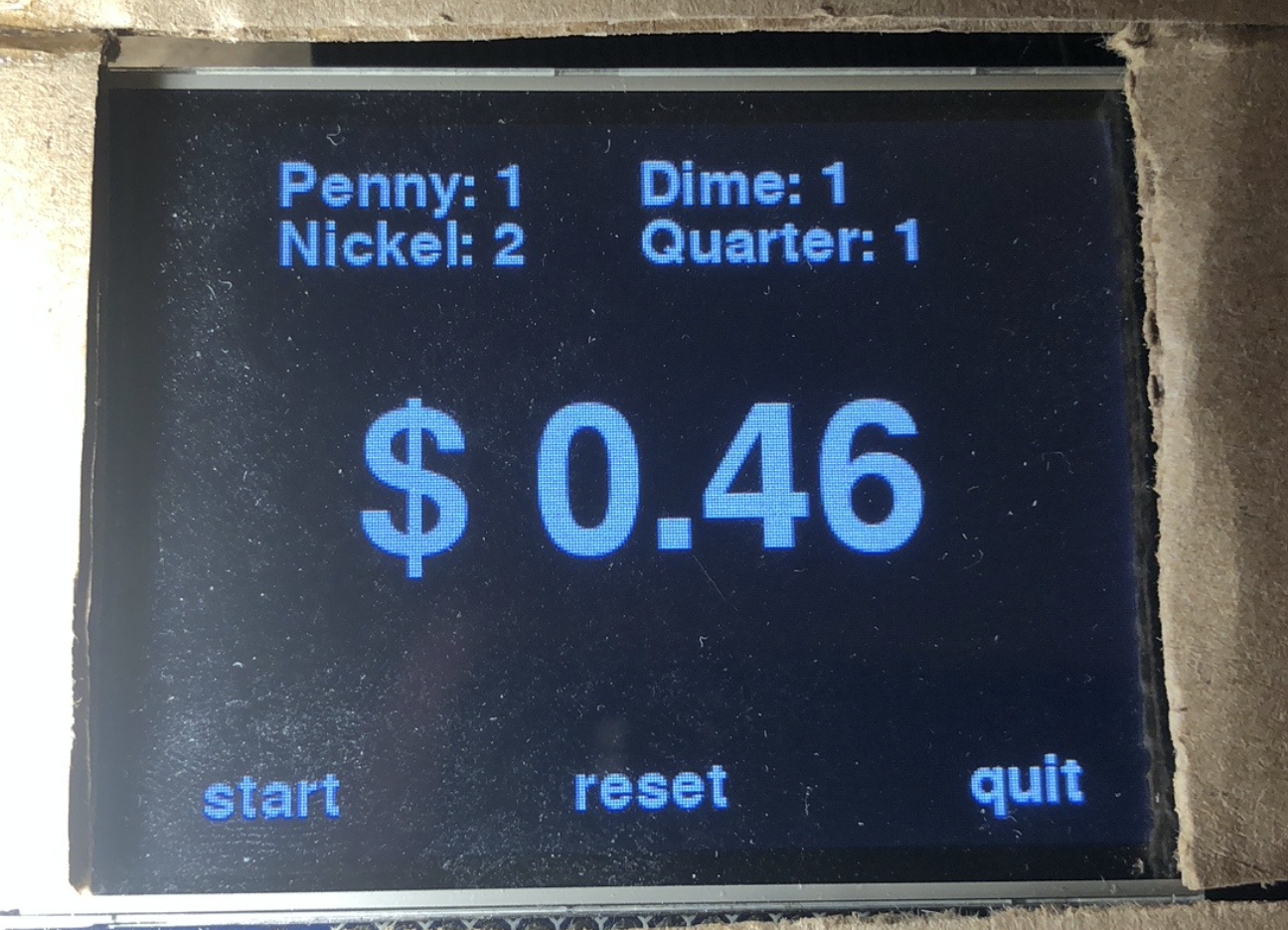## Testing, fine-tuning and result

In testing our coin sorter machine, we are faced with different problems and here is how we approach them to ensure a better result.

• Lighting conditions: It turns out the lighting conditions can have a big effect on our prediction accuracy of the coin types. If lighting is too dark or or shining, the coin in the image won’t have clear edges so that our algorithm will have difficulty detecting the circles. The angle of the lighting source also matters. If we don’t place the lighting source directly above the platform, the coin could have shadow in the image and the circle detected could have a big enough radius to cover the shadow. So in this design it’s vital to have proper lighting conditions to ensure good recognition accuracy.
• Better models to detect coin size: We had two models to detect the coin size of an image and initially we preferred the first model because we believed taking difference between empty image and coin image could well emphasize the pixels within the coin to help HoughCircles() method better identify the circle parameters. However, we neglected the fact that the appearance of a coin could also change the pixels around it (especially pixels closer to the semi-circle paddle) because its shadow might be projected on it. The side effect of this is that the circle area that enclose the coin might get slightly deformed. The HoughCircles() method does not work well in finding the radius of an imperfect circle. So we end up switching to a more straightforward model (model 2). This model also helps us eliminate the need to cache snapshots without a coin on the platform and improve the performance of our application.
• How camera setup affects our predictions: The PiCamera module has several useful parameters. First, we could use the quality argument in capture() or capture_continuous() method to help us get better quality jpeg images (default quality is 80, we change it to 100). A better quality picture will yield better prediction accuracy. Also, we were initially dumping every image out to the hard disk and reading them in, which is not efficient. A better approach is to have an ByteIo buffer store the image content directly, convert them to opencv objects and clear them on taking the next picture.
• How coin feeder cooperates with the robot arm: The speed at which the coin feeder spins matters. If it spins too fast, it might happen that two coins drop onto the platform and there is no way to separate them out (they will be dropped into the same bin). Even if it spins at a reasonable speed, since we are taking multiple photos to make multiple predictions, the spinner could still throw another coin onto the platform while we are taking pictures. As a result, when we detect a coin on the platform, we decide to stop the spinner immediately so that we have enough time to process the current coin. After we finished processing, we will restart the spinner again.
• Make better prediction based on multiple shots: We decided to take multiple snapshots of a single coin and made multiple predictions to ensure we have higher confidence of the prediction result. There is a downside to this, however. We must give the camera some warm-up time before it takes another picture, the warm up time is 0.3-0.4 sec. Since we need at least 4 pictures to finalize the prediction (1 for coin detection, 3 for coin type prediction), it takes at least 1.5 - 2.0 seconds before the robot arm can make a move. We have tried to use the camera’s video port to shorten the warmup time. But it turns out the image quality with this port is too poor to be usable. So we eventually make a compromise to trade time with better prediction accuracy by using the normal port.

## Conclusion

At the end of semester, we were able to deliver a functional coin sorting machine with high accuracy. The Coin Feeder, Image Processing algorithm and robot arm operations work as expected. It's able to carry one coin a time from the Coin Feeder and drop the picked coin to the slope. The coin is able to slide down the slope and stop on top of the platform. The PiCamera is able to take pictures of the coin and our image processing algorithm is able to classify the coin. The robot arm is able to drop the coin to its corresponding collecting bin. The display on PiTFT is informative to users. It clearly displays how many coins have been sorted and the total value of money so far.

## Future Work

We can explore in the future to improve the processing speed of our algorithm. Initially we thought our algorithm could be very fast but it’s actually much slower than expected. The whole image processing algorithm is optimizable if we have more time working on it. There are also some other methods to try out in the future. Overall, we are satisfied with what we have done so far and we are looking forward to optimizing the system in the future.

## Work Distribution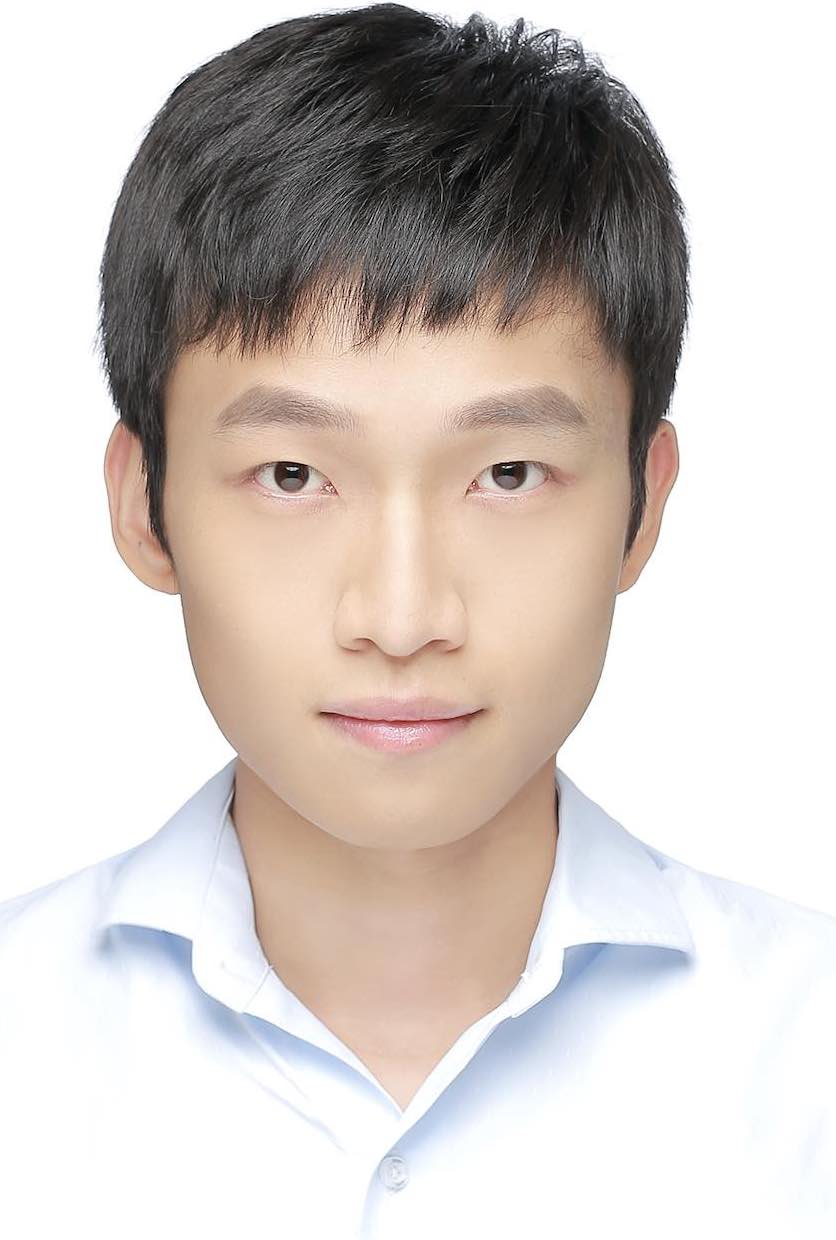### Yidan Wang

yw482@cornell.edu

Designed the software architecture and image processing algorithm### Zhihao Liu

zl385@cornell.edu

Designed the hardware components and some control programs

## References

PiCamera Document
Parallax Continuous Rotation Servo
Pan-Tilt HAT
R-Pi GPIO Document

## Code Appendix

```import io
import os
import time
from time import sleep
import glob
import pickle

import RPi.GPIO as GPIO
import cv2
from pygame.locals import *
import numpy as np
import pygame
from PCA9685 import PCA9685
from picamera import PiCamera
from sklearn.model_selection import train_test_split
from sklearn.neural_network import MLPClassifier

#########################
# Important constants
#########################
IMAGE_CROP_FIRST_DIM = 265, -35
IMAGE_CROP_SECOND_DIM = 290, -250

GPIO.setmode(GPIO.BCM)
GPIO.setup(13, GPIO.OUT)
os.putenv('SDL_VIDEODRIVER', 'fbcon')  # Display on piTFT
os.putenv('SDL_FBDEV', '/dev/fb1')
os.putenv('SDL_MOUSEDRV', 'TSLIB')  # Track mouse clicks on piTFT
os.putenv('SDL_MOUSEDEV', '/dev/input/touchscreen')
pygame.init()
pygame.mouse.set_visible(False)
WHITE = 255, 255, 255
BLACK = 0, 0, 0
screen = pygame.display.set_mode((320, 240))
my_font = pygame.font.Font(None, 30)
my_font_big = pygame.font.Font(None, 100)
my_font_small = pygame.font.Font(None, 20)
my_buttons = {'quit': (280, 220), 'start': (40, 220), 'reset': (160, 220)}

PENNY_TYPE = 1
NICKEL_TYPE = 2
DIME_TYPE = 3
QUARTER_TYPE = 4
EMPTY_TYPE = 5

TYPE_DICT = {
PENNY_TYPE: 'penny',
NICKEL_TYPE: 'nickel',
DIME_TYPE: 'dime',
QUARTER_TYPE: 'quarter',
EMPTY_TYPE: 'empty'
}
PENNY_SAMPLE_FOLDER = 'sample_pic_penny'
DIME_SAMPLE_FOLDER = 'sample_pic_dime'

def generate_sample_coin_size_img(t, nums=30):
folder = PENNY_SAMPLE_FOLDER if t == PENNY_TYPE else DIME_SAMPLE_FOLDER
name_prefix = TYPE_DICT[t]
if not os.path.exists(folder):
os.mkdir(folder)
camera = PiCamera()
cur = glob.glob(r'%s/*' % folder)
start_index = 0 if not cur else 1 + max([int(i[len(folder) + len(name_prefix) + 2:-5]) for i in cur])
for i in range(nums):
stream = io.BytesIO()
camera.capture(stream, format='jpeg', quality=100)
data = np.fromstring(stream.getvalue(), dtype=np.uint8)
image = cv2.imdecode(data, 1)
processed_img_colored = image[IMAGE_CROP_FIRST_DIM:IMAGE_CROP_FIRST_DIM,
IMAGE_CROP_SECOND_DIM:IMAGE_CROP_SECOND_DIM]
processed_img_gray = cv2.cvtColor(processed_img_colored, cv2.COLOR_BGR2GRAY)
circles = cv2.HoughCircles(processed_img_gray, cv2.HOUGH_GRADIENT, dp=3, minDist=50,
if circles is not None and np.max([c for c in circles[0, :]]) >= 20:
x, y, r = np.round(max(circles[0, :], key=lambda i: i)).astype("int")
processed_img_colored_circle = processed_img_colored[y - r:y + r, x - r:x + r, :]
cv2.imwrite('%s/%s-%s.jpeg' % (folder, name_prefix, start_index), processed_img_colored_circle)
start_index += 1

camera.close()

def get_hist(img):
# img is a squared shape

m = np.zeros(img.shape[:2], dtype="uint8")
(w, h) = (int(img.shape / 2), int(img.shape / 2))
cv2.circle(m, (w, h), min(w, h), 255, -1)

h = cv2.calcHist([img], [0, 1, 2], m, [8, 8, 8], [0, 256, 0, 256, 0, 256])

return cv2.normalize(h, h).flatten()

MODEL_FILE_NAME = 'penny_or_dime_model.sav'
if os.path.exists(MODEL_FILE_NAME):
clf = MLPClassifier(solver="lbfgs")
X = []
y = []
for t in [PENNY_TYPE, DIME_TYPE]:
folder = PENNY_SAMPLE_FOLDER if t == PENNY_TYPE else DIME_SAMPLE_FOLDER
for i in glob.glob('%s/*.jpeg' % folder):
y.append(t)

print('Length of sample:', len(X))
X_train, X_test, y_train, y_test = train_test_split(
X, y, test_size=.2)
clf.fit(X_train, y_train)
score = int(clf.score(X_test, y_test) * 100)
print("MLPClassifier accuracy: ", score)
pickle.dump(clf, open(MODEL_FILE_NAME, 'wb'))
return clf

def get_dis(v1, v2):
return np.sum((v1 - v2) ** 2)

def change(p, pulse, sleep_time):
# print "Change pulse to %s" % str(pulse)
freq, dc = get_freq_dc(pulse)
p.ChangeFrequency(freq)
p.ChangeDutyCycle(dc)
sleep(sleep_time)

def get_freq_dc(pulse):
return 1000.0 / (pulse + 20), pulse / (20.0 + pulse) * 100

def draw(penny=0, nickel=0, dime=0, quarter=0):
screen.fill(BLACK)  # Erase the Work space
for my_text, text_pos in my_buttons.items():
text_surface = my_font.render(my_text, True, WHITE)
rect = text_surface.get_rect(center=text_pos)
screen.blit(text_surface, rect)

text_box = my_font.render('Penny: %d' % penny, True, WHITE)
rect = text_box.get_rect(topleft=(40, 10))
screen.blit(text_box, rect)
text_box = my_font.render('Nickel: %d' % nickel, True, WHITE)
rect = text_box.get_rect(topleft=(40, 30))
screen.blit(text_box, rect)
text_box = my_font.render('Dime: %d' % dime, True, WHITE)
rect = text_box.get_rect(topleft=(160, 10))
screen.blit(text_box, rect)
text_box = my_font.render('Quarter: %d' % quarter, True, WHITE)
rect = text_box.get_rect(topleft=(160, 30))
screen.blit(text_box, rect)
total = 0.01 * penny + nickel * 0.05 + dime * 0.1 + quarter * 0.25
text_box = my_font_big.render('\$ %.2f' % total, True, WHITE)
rect = text_box.get_rect(center=(160, 120))
screen.blit(text_box, rect)

pygame.display.flip()

class CoinSorter:
def __init__(self, debug_mode=False):

self.debug_mode = debug_mode
self.penny = 0
self.nickel = 0
self.dime = 0
self.quarter = 0
self.update_UI()

self.die = False

self.snapshot_counter = 0

self.robot = PCA9685()

# initialize spinner (not spinning yet)
freq, dc = get_freq_dc(1.5)
self.spinner = GPIO.PWM(13, freq)
self.spinner.start(0.0)

self.robot.setPWMFreq(50)
self.robot.setRotationAngle(1, 90)
self.robot.setRotationAngle(0, 20)

self.camera = PiCamera()

# camera warm-up
sleep(2)

self.t1.start()

def update_UI(self):
draw(penny=self.penny, nickel=self.nickel, dime=self.dime, quarter=self.quarter)

def start_sorter(self):
self.start_spinner()
self.start_job()

def preprocess_img(self, img, gray=True):
processed = img[IMAGE_CROP_FIRST_DIM:IMAGE_CROP_FIRST_DIM,
IMAGE_CROP_SECOND_DIM:IMAGE_CROP_SECOND_DIM]
return cv2.cvtColor(processed, cv2.COLOR_BGR2GRAY) if gray else processed

def take_snapshot(self, use_video_port=False, quality=100):
stream = io.BytesIO()
self.snapshot_counter += 1
self.camera.capture(stream, format='jpeg', use_video_port=use_video_port, quality=quality)
data = np.fromstring(stream.getvalue(), dtype=np.uint8)
return cv2.imdecode(data, 1)

def take_continuous_snapshots(self, use_video_port=False, quality=100):
stream = io.BytesIO()
for _ in self.camera.capture_continuous(stream, format='jpeg', use_video_port=use_video_port, quality=quality):
stream.truncate()
stream.seek(0)
self.snapshot_counter += 1
data = np.fromstring(stream.getvalue(), dtype=np.uint8)
yield cv2.imdecode(data, 1)

def detect_coin_hough_circles(self, processed_img):
circles = self.hough_circles(processed_img)
return circles and np.max([c for c in circles[0, :]]) > 20

def hough_circles(self, img):

def reset(self):
self.penny = 0
self.nickel = 0
self.dime = 0
self.quarter = 0

def start_job(self):

while not self.die:

for img in self.take_continuous_snapshots():
if self.get_coin_type(img) != EMPTY_TYPE:
break
if self.die:
return
self.stop_spinner()
counter = {}
for img in self.take_continuous_snapshots():
t = self.get_coin_type(img)

if t not in counter:
counter[t] = 0
counter[t] += 1
if t == EMPTY_TYPE:
if counter[t] >= 3:
break
elif counter[t] == 3:
self.increment_type_count(t)
self.put_coin(t)
self.update_UI()
break
self.start_spinner()

def increment_type_count(self, t):
if t == PENNY_TYPE:
self.penny += 1
elif t == NICKEL_TYPE:
self.nickel += 1
elif t == QUARTER_TYPE:
self.quarter += 1
elif t == DIME_TYPE:
self.dime += 1
else:
raise RuntimeError("Wrong type passed")

def get_coin_type(self, raw_img):

processed_img_colored = self.preprocess_img(raw_img, gray=False)
processed_img_gray = cv2.cvtColor(processed_img_colored, cv2.COLOR_BGR2GRAY)
circles = self.hough_circles(processed_img_gray)
t = EMPTY_TYPE
if circles is not None and np.max([c for c in circles[0, :]]) >= 20:
x_f, y_f, r_f = max(circles[0, :], key=lambda i: i)
if self.debug_mode:
print(r_f)
if r_f >= 40:
t = QUARTER_TYPE
else:
x, y, r = np.round(max(circles[0, :], key=lambda i: i)).astype("int")
t = self.model_penny_or_dime.predict([get_hist(processed_img_colored[y - r:y + r, x - r:x + r, :])])
if t != PENNY_TYPE:
t = NICKEL_TYPE if r_f > 34.5 else DIME_TYPE

if self.debug_mode:
print(TYPE_DICT[t])

return t

def put_coin(self, t):
if t == PENNY_TYPE:
self.dump_coin(0)
elif t == NICKEL_TYPE:
self.dump_coin(60)
elif t == DIME_TYPE:
self.dump_coin(120)
else:
self.dump_coin(180)

def dump_coin(self, angle):
self.robot.setRotationAngle(1, angle)
time.sleep(0.2)
self.robot.setRotationAngle(0, 120)
time.sleep(0.5)
self.robot.setRotationAngle(0, 20)
time.sleep(0.2)
self.robot.setRotationAngle(1, 90)

def start_spinner(self):
change(self.spinner, 1.54, 0.0)

def stop_spinner(self):
change(self.spinner, 0.0, 0.0)

def shutdown(self):
self.die = True
self.t1.join()
self.robot.setRotationAngle(1, 90)
self.robot.setRotationAngle(0, 20)
self.robot.exit_PCA9685()
self.stop_spinner()
self.spinner.stop()
self.camera.close()

coin_sorter = CoinSorter()
exit_program = False
coin_sorter_start = False

try:
while not exit_program:
time.sleep(0.02)
for event in pygame.event.get():
if (event.type is MOUSEBUTTONDOWN):
pos = pygame.mouse.get_pos()
elif (event.type is MOUSEBUTTONUP):
pos = pygame.mouse.get_pos()
x, y = pos
if y > 180:
if x >= 212:
exit_program = True
elif x < 212 and x > 106:
coin_sorter.reset()
coin_sorter.update_UI()
else:
if not coin_sorter_start:
coin_sorter_start = True